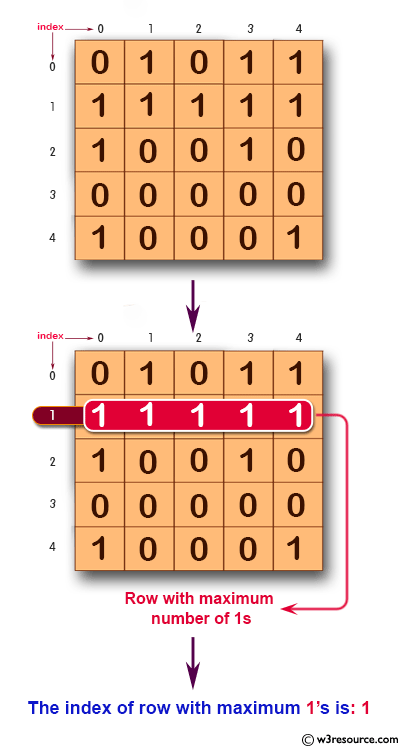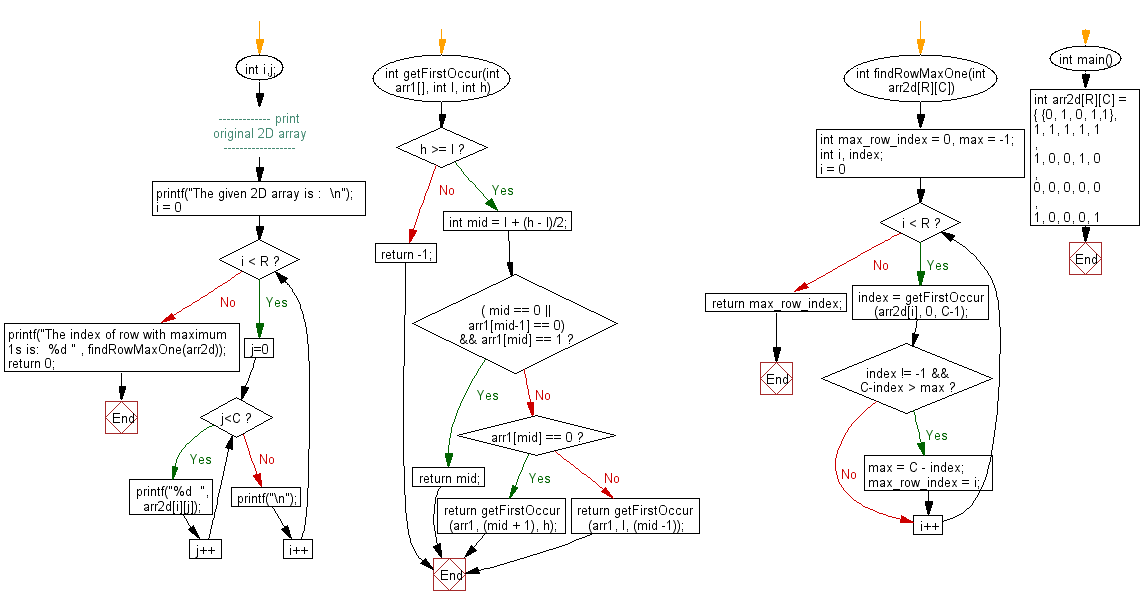﻿ C exercises: Find the row with maximum number of 1s - w3resource# C Exercises: Find the row with maximum number of 1s

## C Array: Exercise-60 with Solution

Write a program in C to find the row with maximum number of 1s.

Pictorial Presentation:Sample Solution:

C Code:

``````#include <stdio.h>
#define R 5
#define C 5

int getFirstOccur(int arr1[], int l, int h)
{
if(h >= l)
{
int mid = l + (h - l)/2;
if ( ( mid == 0 || arr1[mid-1] == 0) && arr1[mid] == 1)
return mid;
else if (arr1[mid] == 0)
return getFirstOccur(arr1, (mid + 1), h);
else
return getFirstOccur(arr1, l, (mid -1));
}
return -1;
}
int findRowMaxOne(int arr2d[R][C])
{
int max_row_index = 0, max = -1;
int i, index;
for (i = 0; i < R; i++)
{
index = getFirstOccur (arr2d[i], 0, C-1);
if (index != -1 && C-index > max)
{
max = C - index;
max_row_index = i;
}
}
return max_row_index;
}

int main()
{
int arr2d[R][C] = { {0, 1, 0, 1,1},
{1, 1, 1, 1, 1},
{1, 0, 0, 1, 0},
{0, 0, 0, 0, 0},
{1, 0, 0, 0, 1}
};
int i,j;
//------------- print original 2D array ------------------
printf("The given 2D array is :  \n");
for(i = 0; i < R; i++)
{
for(j=0; j<C ; j++)
{
printf("%d  ", arr2d[i][j]);
}

printf("\n");
}
//------------------------------------------------------
printf("The index of row with maximum 1s is:  %d " , findRowMaxOne(arr2d));
return 0;
}
```
```

Sample Output:

```The given 2D array is :
0  1  0  1  1
1  1  1  1  1
1  0  0  1  0
0  0  0  0  0
1  0  0  0  1
The index of row with maximum 1s is:  1
```

Flowchart:C Programming Code Editor:

Improve this sample solution and post your code through Disqus.

What is the difficulty level of this exercise?

﻿